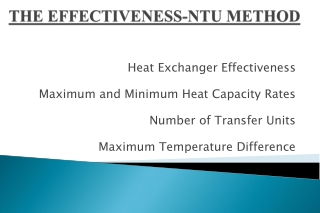# THE EFFECTIVENESS-NTU METHOD - PowerPoint PPT PresentationDownload PresentationTHE EFFECTIVENESS-NTU METHOD

Download Presentation## THE EFFECTIVENESS-NTU METHOD

- - - - - - - - - - - - - - - - - - - - - - - - - - - E N D - - - - - - - - - - - - - - - - - - - - - - - - - - -
##### Presentation Transcript

1. THE EFFECTIVENESS-NTU METHOD Heat Exchanger Effectiveness Maximum and Minimum Heat Capacity Rates Number of Transfer Units Maximum Temperature Difference

2. Effectiveness, ε • LMTD application is easy when the temperatures are known • When the outlet temperatures are unknown tedious iterations are required to solve problems • Kays & London developed the Effectiveness-NTU • Method for simplifying heat exchanger analysis:

3. Heat Capacity Rates • Qmax = the heat transfer rate achievable were it possible to heat the lower heat capacity stream to the inlet temperature of the other. • Qactual (NRG bal) = Cc(Tc,out – Tc,in) = Ch(Th,in – Th,out) • Cc = mcCpcand Ch = mhCphThe heat capacity rates of the cold and hot streams, respectively

4. ΔTmax, Qmax & Cmin • ΔTmax=Th,in –Tc,in ( max temp. diff. is hot fluid-cold fluid inlet temp. diff.) • Maximum heat transfer • Cold fluid heated to inlet temp of hot fluid • Hot fluid cooled to inlet temp of cold fluid • Fluid with smaller heat capacity rate has larger temp. change. Qmax = Cmin(Th,in – Tc,in)

5. Examples • (1)A flow of 1.0 kg/s of an organic liquid of heat capacity 2.0 kJ/kg K is cooled from 350.0 K to 330.0 K by a stream of water flowing counter currently through a double pipe heat exchanger. Estimate the effectiveness of the unit if the water enters the exchanger at 290.0 K. • (2) Cold water enters a counter flow heat exchanger at 10.0 oC at a rate of 8.0 kg/s where it is heated by a hot water stream that enters the heat exchanger at 70.0 oC at a rate of 2.0 kg/s. Assuming the specific heat capacity of water to be constant at Cp=4.18 kJ/kg oC, determine the maximum heat transfer rate and the outlet temperatures of the cold and hot water streams.

6. Effectivenes • Since • So, with the effectiveness of the heat exchanger we can determine the heat transfer rate without knowing the outlet temperatures. • Effectiveness is a function of heat exchanger geometry and flow arrangement

7. ε, For Double Pipe in Parallel Flow • Recall from alternate method of deriving LMTD • For a parallel flow H/E. Also, from Qactual: • Substituting this into equation at the top and adding & subtracting Tc,1 gives:

8. Which simplifies to: Manipulating the effectiveness defining equation gives: Substituting this result into the equation at the top and solving for ε gives the following relation for the effectiveness of a parallel flow heat exchanger:

9. Taking either Cc or Ch to be Cmin gives: UAs/Cmin is a dimensionless group called Number of Transfer Units or NTU, where:

10. Capacity Ratio Heat exchanger effectiveness is a function of both NTU and capacity ratio Kays and London investigated and developed effectiveness and capacity ratios for many heat exchanger arrangements and made the results available in chart form Eg. Repeat the last problem where the oil flow rate was halved, using the effectiveness method.

11. Parallel & Counter Flow Charts

12. Charts for Multi pass flows

13. Unmixed Fluids

14. Effectiveness and NTU Relations • For greater precision the charts not very userfriendly • For computer based analyses expressions of the curves would be more applicable • Expressions were developed for both NTU and ε relations

15. Effectiveness Double pipe parallel flow counter flow counter flow, c=1

16. NTU Cross flow: cmax mixed, cmin unmixed cmax unmixed, cmin mixed Shell & tube one shell pass 2,4,6, tube passes All exchangers, c=0

17. Example #3 • A process requires a flow of 4.0 kg/s of purified water at 340.0 K to be heated from 320.0 K by 8.0 kg/s of untreated water which can be available at 380.0, 370.0, 360.0, or 350.0 K. Estimate the heat transfer surfaces of one shell pass two tube pass heat exchangers that are suitable for these duties. In all cases the mean heat capacity of the water is 4.18 kJ/kg K. bn

18. Option of one large vs. two small H/E • If surface area is the major concern for H/E selection can two half sized H/E suffice for one large one? • Example#4 : A counter flow concentric tube H/E is used to heat 1.25 kg/s of water from 35.0 K to 80.0 K by cooling an oil with cP=2.0 kJ/kg K from 150.0 K to 85.0 K. The overall U= 850.0 kW/m2 K. A similar arrangement is to be built at another plant but for comparison, two smaller counter flow H/Es connected in series on the water side and in parallel on the oil side are contemplated. If the flow is split equally between the two H/Es and the overall U is the same for all the H/Es but the smaller H/Es cost more per unit surface area, which arrangement would be the more economical; the larger or the two equally sized H/Es ?

19. Toil 1=150K H/Exchanger 1 H/Exchanger 2 Tw1=35 K Tw2 Tw3=80K Toil 2,1 Toil 22 Toil 2=85K